Function Repository Resource:

# RecurringDecimalForm

Display a number in decimal form so that recurring digit sequences are highlighted and displayed only once

Contributed by: Jon McLoone
 ResourceFunction["RecurringDecimalForm"][val] displays numeric value val such that recurring digits are highlighted and displayed only once. ResourceFunction["RecurringDecimalForm"][val,b] displays numeric value val such that digits recurring in base b are highlighted and displayed only once.

## Details and Options

ResourceFunction["RecurringDecimalForm"] takes the following options:
 "MinRepeats" 3 number of times digits must repeat before they are assumed to be recurring "RoundingDigits" 1 number assumed to be affected by rounding and, as such, ignored "Notation" "Vinculum" display notation to be used for recurring digits; choices are "Vinculum", "Dots", "Parenthesis" or "Arc"

## Examples

### Basic Examples (2)

Some rational numbers have recurring decimal digits:

 In:=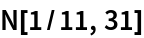Out=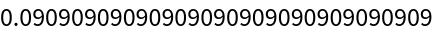RecurringDecimalForm displays this in a more compact notation:

 In:=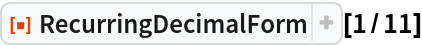Out=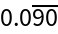### Scope (5)

RecurringDecimalForm accepts any input that is numeric:

 In:=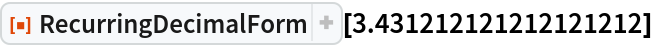Out=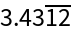RecurringDecimalForm ignores repeating zeros:

 In:=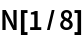Out=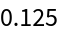In:=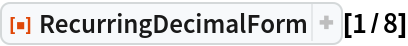Out=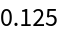For numeric quantities, a repeating sequence in the final digits (excluding the last digit, which is subject to rounding) is assumed to be recurring:

 In:=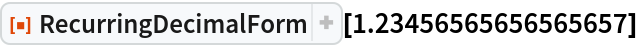Out=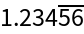For exact numeric quantities that are not explicitly Rational, RecurringDecimalForm searches the first \$MaxExtraPrecision digits:

 In:=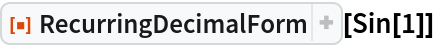Out=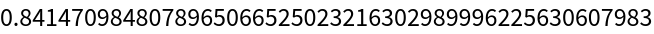Recurring digits can be found in other number bases:

 In:=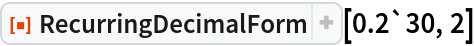Out=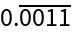### Options (6)

#### MinRepeats (2)

Digit sequences that repeat at least three times are assumed to be recurring:

 In:=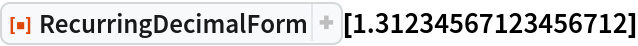Out=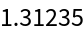The option "MinRepeats" controls this sensitivity:

 In:=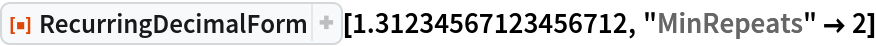Out=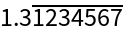#### Notation (1)

There are several notations for the display of recurring digits used in different parts of the world:

 In:=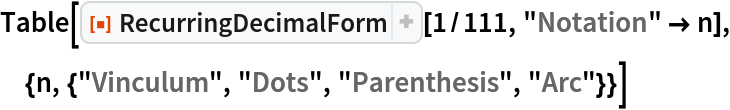Out=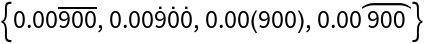#### RoundingDigits (3)

By default, the final digit is assumed to be subject to rounding and is ignored:

 In:=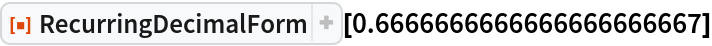Out=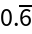In some circumstances, more digits are affected by rounding:

 In:=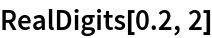Out=In:=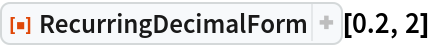Out=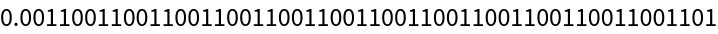You can ignore more digits with "RoundingDigits":

 In:=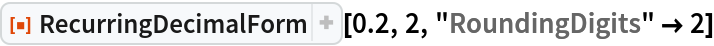Out=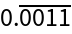### Possible Issues (4)

By default, the final digit is assumed to be subject to rounding and is ignored:

 In:=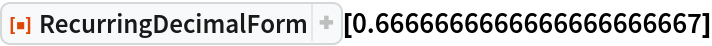Out=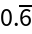In:=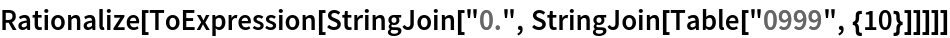Out=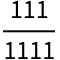In some circumstances, more digits are affected by rounding:

 In:=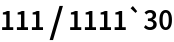Out=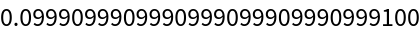In these circumstances, RecurringDecimalForm will not recognize the repeat:

 In:=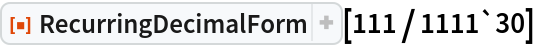Out=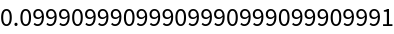You can ignore more digits with "RoundingDigits":

 In:=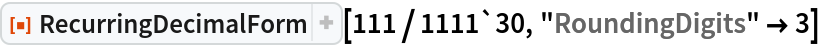Out=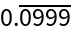Jon McLoone

## Version History

• 1.0.1 – 08 April 2022
• 1.0.0 – 06 December 2019